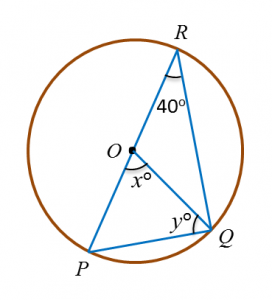3.2.2 Circles II, PT3 Practice

3.2.2 Circles II, PT3 Practice

Question 6:
Diagram below shows a circle with centre O. POR is a straight line.Find the value of x and of y.

Solution:
$\begin{array}{l}x=40×2\\ \text{}=80\\ \\ y=\frac{180-80}{2}\\ \text{}=50\end{array}$

Question 7:
Diagram below shows a circle with centre O.Find the value of x.

Solution:
$\begin{array}{l}\angle QRO={38}^{o},\angle ORS={44}^{o}\\ \angle QRS={38}^{o}+{44}^{o}={82}^{o}\\ {x}^{o}={82}^{o}×2\\ {x}^{o}={164}^{o}\\ x=164\end{array}$

Question 8:
Diagram below shows a circle. QTS is the diameter of the circle and PTR is a straight line.Find the value of x.

Solution:
$\begin{array}{l}\angle RPS={90}^{o}-{42}^{o}={48}^{o}\\ {x}^{o}={48}^{o}+{37}^{o}={85}^{o}\end{array}$

Question 9:
Diagram below shows a circle with centre O. PQR is a straight line.Find the value of x and of y.

Solution:
Angle at Q = 180o – 70o = 110o
x2 = exterior angle at Q
= 180o – 110o
= 70o
x = 70

yo = reflex angle at O
yo = 360o – (70o × 2)
yo = 220o
y = 220

Question 10:
In diagram below, ABCD and DEFG are straight lines.Find the value of x and of y.

Solution: